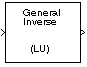# LU Inverse

Compute inverse of square matrix using LU factorization

## Library

Math Functions / Matrices and Linear Algebra / Matrix Inverses

`dspinverses`## Description

The LU Inverse block computes the inverse of the square input matrix A by factoring and inverting row-pivoted variant Ap.

`${A}_{p}^{-1}={\left(LU\right)}^{-1}$`

L is a lower triangular square matrix with unity diagonal elements, and U is an upper triangular square matrix. The block outputs the inverse matrix A-1.

## Examples

See Matrix Inverses for an example that uses the LU Inverse block.

## References

Golub, G. H., and C. F. Van Loan. Matrix Computations. 3rd ed. Baltimore, MD: Johns Hopkins University Press, 1996.

## Supported Data Types

• Double-precision floating point

• Single-precision floating point

 Cholesky Inverse DSP System Toolbox LDL Inverse DSP System Toolbox LU Factorization DSP System Toolbox LU Solver DSP System Toolbox `inv` MATLAB

See Matrix Inverses for related information.

Watch now